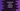# JavaScript array values() function## JavaScript array values() function:

The values() is an inbuilt function of JavaScript array. By using this function, we can get a new array iterator object that holds the array elements.

In this post, we will learn the definition of values function and how to use it with different examples.

### Definition of values:

The values() function is defined as like below:

``values()``

It doesn’t take any argument. Since this is an inbuilt method, you can call it on any JavaScript array.

#### Return value of values():

The values() function returns a new array iterator object.

### Example of values():

Since this function returns a new array iterable object, we can use it to iterate over the items of an array.

Let me show you an example:

``````let arr = ['hello', 'world', '!!'];

let iterator = arr.values();

for(let e of iterator){
console.log(e);
}``````

Here,

• arr is the given array.
• values() function is called on this array.
• The iterator returned by values() function is stored in the iterator variable.
• The for of loop iterates over the contents of iterator and prints the values.
• The console.log is printing each value.

If you run this program, it will print the below output:

``````hello
world
!!``````

Or, you can use it directly:

``````let arr = ['hello', 'world', '!!'];

for(let e of arr.values()){
console.log(e);
}``````

### Example using next():

Let’s try it with next(). We can print the value property of the return value of next() to print the iterator content.

``````let arr = ['hello', 'world', '!!'];

let iterator = arr.values();

console.log(iterator.next().value);
console.log(iterator.next().value);
console.log(iterator.next().value);``````

It will print:

``````hello
world
!!``````

### Note: Iterator is temporary:

The iterator created is a temporary object. Once the iteration is done, if you try to iterate over it again, it will give undefined.

For example:

``````let arr = ['hello', 'world', '!!'];

let iterator = arr.values();

for(let e of iterator){
console.log(e);
}

for(let e of iterator){
console.log(e);
}``````

This is the same example we tried above. But, we are using the for..of loop two times with the iterator in this example.

It will print:

``````hello
world
!!``````

As you can see, the second loop doesn’t work.

You need to get an iterator each time you are iterating over it.

``````let arr = ['hello', 'world', '!!'];

for(let e of arr.values()){
console.log(e);
}

for(let e of arr.values()){
console.log(e);
}``````

Both loops work in this program because we are creating a new iterator.

Again, if you use next,

``````let arr = ['hello', 'world', '!!'];

let iterator = arr.values();

console.log(iterator.next().value);
console.log(iterator.next().value);
console.log(iterator.next().value);
console.log(iterator.next().value);
console.log(iterator.next().value);``````

The first three will print the contents of the array and once it is done, it will print undefined:

``````hello
world
!!
undefined
undefined``````

### Note: Iterator stores the address:

When we create an iterator, the items of the array are not stored in that iterator. Instead, it stores the address of the array that calls values(). So, if the array content changed in between, the iterator will return the new value.

For example:

``````let arr = ['hello', 'world', '!!'];

let iterator = arr.values();

console.log(iterator.next().value);

arr = 'Universe';
arr = '😃';

console.log(iterator.next().value);
console.log(iterator.next().value);``````

It will print:

``````hello
Universe
😃``````

After the first console.log, we changed the second and the third items of the array. So, it printed the new values of the array.